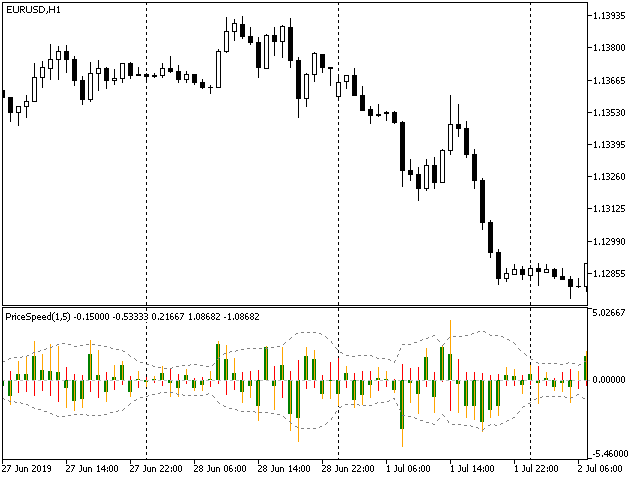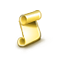Interesting script?
So post a link to it -
let others appraise it

You liked the script? Try it in the MetaTrader 5 terminal# PriceSpeed - indicator for MetaTrader 5

Views:
3128
Rating:
Published:
2019.07.07 22:25

The indicator shows the speed of price change in points per minute. The general idea is taken from the Average Speed indicator, but the code has been improved, and the functionality has been expanded. In addition to the average price speed, which may be interesting for evaluation of trends, the peak speed has been added to the new indicator, which allows implementing breakout and pulse strategies.

Estimation of the peak speed is found based on the difference between high and low prices of each candle (this is the maximum distance that the price covers for the duration of the timeframe). This is an estimate, not an exact value, and the estimate is the lower bound, since in most cases, time passed between high and low moments is much less than duration of the candle (timeframe), that is, the speed is actually higher. Smaller timeframes are not analyzed by the indicator.

In addition to the real peak speed in the direction of the candle, the indicator calculates false peak speeds, which are formed during the movements from open to first extremum (high or low, depeding from candle configuration) and from second extremum to close - both in the direction opposite to the real type of the candle.

Thus, the indicator displays three buffers as histograms:

• average price speed according to the algorithm of the original indicator (thick green);
• peak real price speed (thin orange);
• peak false price rate (thin red);

To facilitate decision making (how important are real and false peak speeds), the indicator additionally calculates the standard deviation of peak speeds for a given number of bars - it is displayed as a corridor of dotted lines.### Parameters

• Averaging Bars - the number of bars for averaging the speed of prices; both the original speed and peak ones are averaged (although when working with peak speeds, it makes sense not to do averaging, that is, to use 1);
• Price - price type for calculating the speed (it makes sense only for the original average price speed; not used for peak speeds);
• Peak Deviation Period - the number of bars for calculating the standard deviation of peak speeds;RSI candles - lite ressourceSmoothed CCIUnityExport Calculated Prices & Indicator Buffers